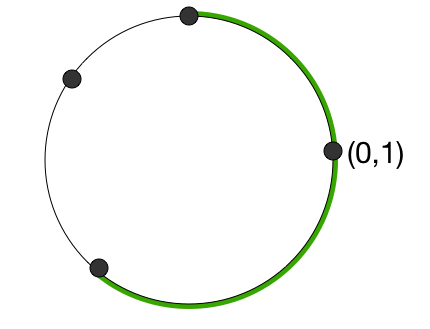# Points On A Circle

Probability Level 4Consider the unit circle $x^2 + y^ 2 = 1$.
Choose 3 points uniformly at random on the circumference, which divides the circle into 3 arcs.

What is the expected length of the arc that contains the point $(1,0)$?

Technical details: We pick a point on the circumference uniformly at random in the following manner.
1. First select $\theta \sim U[0,1]$, the uniform distribution on the unit interval
2. Next, we pick the point $p = \left( \cos ( 2 \pi \theta) , \sin ( 2 \pi \theta) \right)$.

×